# North West Corner Rule

The North West corner rule is a method for computing a basic feasible solution of a transportation problem, where the basic variables are selected from the North – West corner ( i.e., top left corner ).

The standard instructions for a transportation model are paraphrased below. Please read them carefully.

##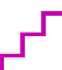Steps in North West Corner Rule

1. Select the upper left-hand corner cell of the transportation table and allocate as many units as possible equal to the minimum between available supply and demand, i.e., min(s1, d1).
2. Adjust the supply and demand numbers in the respective rows and columns.
3. If the demand for the first cell is satisfied, then move horizontally to the next cell in the second column.
4. If the supply for the first row is exhausted, then move down to the first cell in the second row.
5. If for any cell, supply equals demand, then the next allocation can be made in cell either in the next row or column.
6. Continue the process until all supply and demand values are exhausted.

This trial routing method is often far from optimal.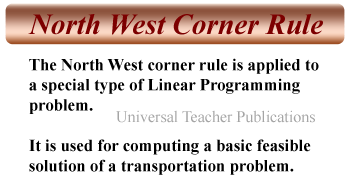Example-1, Example-2

###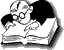Example 1: North West Corner Rule - Transportation Problem

The Amulya Milk Company has three plants located throughout a state with production capacity 50, 75 and 25 gallons. Each day the firm must furnish its four retail shops R1, R2, R3, & R4 with at least 20, 20 , 50, and 60 gallons respectively. The transportation costs (in Rs.) are given below.

Plant Retail Shop Supply
R1 R2 R3 R4
P1 3 5 7 6 50
P2 2 5 8 2 75
P3 3 6 9 2 25
Demand 20 20 50 60

The economic problem is to distribute the available product to different retail shops in such a way so that the total transportation cost is minimum

Solution.

Starting from the North west corner, we allocate min (50, 20) to P1R1, i.e., 20 units to cell P1R1. The demand for the first column is satisfied. The allocation is shown in the following table.

Table 1

On small screens, scroll horizontally to view full calculation

Plant Retail Shop Supply
R1 R2 R3 R4
P1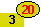5 7 6 50  30
P2 2 5 8 2 75
P3 3 6 9 2 25
Demand 20 20 50 60

Now we move horizontally to the second column in the first row and allocate 20 units to cell P1R2. The demand for the second column is also satisfied.

Table 2

Plant Retail Shop Supply
R1 R2 R3 R4
P1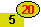7 6 50  30 10
P2 2 5 8 2 75
P3 3 6 9 2 25
Demand 20 20 50 60

Proceeding in this way, we observe that P1R3 = 10, P2R3 = 40, P2R4 = 35, P3R4 = 25. The resulting feasible solution is shown in the following table.

Final Table

Use Horizontal Scrollbar to View Full Table Calculation

Plant Retail Shop Supply
R1 R2 R3 R4
P1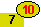6 50
P2 2 5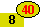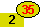75
P3 3 6 9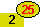25
Demand 20 20 50 60

Here, number of retail shops(n) = 4, and
Number of plants (m) = 3

Number of basic variables = m + n – 1 = 3 + 4 – 1 = 6.

#### Initial basic feasible solution

The total transportation cost is calculated by multiplying each xij in an occupied cell with the corresponding cij and adding as follows:

20 X 3 + 20 X 5 + 10 X 7 + 40 X 8 + 35 X 2 + 25 X 2 = 670

Share this article with your friends

##### Menu
•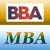MBA & BBA
•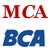BCA & MCA
•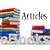Ebooks & Articles
•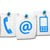Contact us